JEE  >  Chemical Equilibrium MCQ - 1 (Advanced)

# Chemical Equilibrium MCQ - 1 (Advanced)

Test Description

## 25 Questions MCQ Test Chemistry for JEE Advanced | Chemical Equilibrium MCQ - 1 (Advanced)

Chemical Equilibrium MCQ - 1 (Advanced) for JEE 2022 is part of Chemistry for JEE Advanced preparation. The Chemical Equilibrium MCQ - 1 (Advanced) questions and answers have been prepared according to the JEE exam syllabus.The Chemical Equilibrium MCQ - 1 (Advanced) MCQs are made for JEE 2022 Exam. Find important definitions, questions, notes, meanings, examples, exercises, MCQs and online tests for Chemical Equilibrium MCQ - 1 (Advanced) below.
Solutions of Chemical Equilibrium MCQ - 1 (Advanced) questions in English are available as part of our Chemistry for JEE Advanced for JEE & Chemical Equilibrium MCQ - 1 (Advanced) solutions in Hindi for Chemistry for JEE Advanced course. Download more important topics, notes, lectures and mock test series for JEE Exam by signing up for free. Attempt Chemical Equilibrium MCQ - 1 (Advanced) | 25 questions in 60 minutes | Mock test for JEE preparation | Free important questions MCQ to study Chemistry for JEE Advanced for JEE Exam | Download free PDF with solutions
 1 Crore+ students have signed up on EduRev. Have you?
*Multiple options can be correct
Chemical Equilibrium MCQ - 1 (Advanced) - Question 1

### Which of the following statements is (are) correct ?

*Multiple options can be correct
Chemical Equilibrium MCQ - 1 (Advanced) - Question 2

### The value of equilibrium constant of a reversible reaction at a given temperature :

Detailed Solution for Chemical Equilibrium MCQ - 1 (Advanced) - Question 2 Equilibrium constant is independent of original concentration of reactant.
*Multiple options can be correct
Chemical Equilibrium MCQ - 1 (Advanced) - Question 3

### Which of the following statement is (are) correct ?

*Multiple options can be correct
Chemical Equilibrium MCQ - 1 (Advanced) - Question 4

If is found that the equilibrium constant increases by factor of four when the temperature is increased from 25°C to 40°C. The value of ΔH° is

Detailed Solution for Chemical Equilibrium MCQ - 1 (Advanced) - Question 4

As we know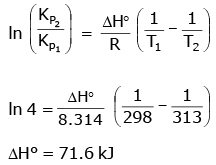*Multiple options can be correct
Chemical Equilibrium MCQ - 1 (Advanced) - Question 5

Ammonium carbamate dissociates as follows :

NH2COONH4(s)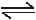2NH3(g) + CO2(g)

The value of KP for this reaction is found to be equal to 2.92 × 10-5 atm3. If one mole of ammonium carbamate is heated in a sealed container, the total pressur develped in the container is

Detailed Solution for Chemical Equilibrium MCQ - 1 (Advanced) - Question 5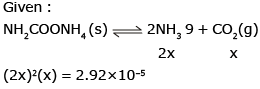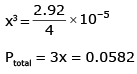*Multiple options can be correct
Chemical Equilibrium MCQ - 1 (Advanced) - Question 6

The equilibrium constant for the following reactions at 1400 K are given.

2H2O(g)2H2(g) + O2(g) ; K1=2.1×10-13

2CO2(g)2CO(g)+O2(g);K2 = 1.4 × 10-12

Then, the equilibrium constant K for the reaction

H2(g) + CO2(g)CO(g) + H2O(g) is

Detailed Solution for Chemical Equilibrium MCQ - 1 (Advanced) - Question 6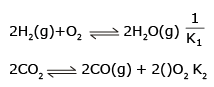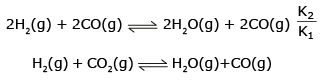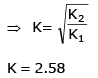*Multiple options can be correct
Chemical Equilibrium MCQ - 1 (Advanced) - Question 7

Under what conditions of temperature and pressure, the formation of atomic hydrogen from molecular hydrogen will be favoured most ?

Detailed Solution for Chemical Equilibrium MCQ - 1 (Advanced) - Question 7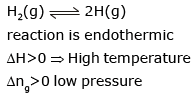*Multiple options can be correct
Chemical Equilibrium MCQ - 1 (Advanced) - Question 8

For the reaction PCl5(g)PCl3(g) + Cl2(g), the forward reaction at constant temperature is favoured by

Detailed Solution for Chemical Equilibrium MCQ - 1 (Advanced) - Question 8

Introduction of inet gas at constant Pressure will increase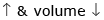Pressure of gases of equilibrium
⇒ equilibrium shifted forward

*Multiple options can be correct
Chemical Equilibrium MCQ - 1 (Advanced) - Question 9

When NaNO3(s) is heated in a closed vessel, oxygen is liberated and NaNO2(s) is left behind. At equilibrium.

Detailed Solution for Chemical Equilibrium MCQ - 1 (Advanced) - Question 9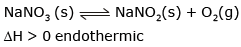High temperature
∴  forward reaction favoured 1000 pressure
High pressure → Reverse reaction

*Multiple options can be correct
Chemical Equilibrium MCQ - 1 (Advanced) - Question 10

Phase diagram of CO2 is shown as following

Based on above find the correct statement (s)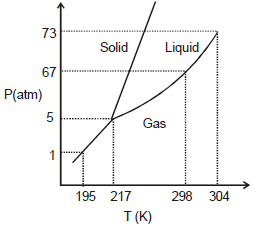*Multiple options can be correct
Chemical Equilibrium MCQ - 1 (Advanced) - Question 11

The equilibrium between, gaseous isomers A, B and C can be represented as

Reaction                                           Equilibrium constant

A(g)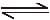B (g)                            : K1 = ?

B(g)C(g)                             : K2 = 0.4

C(g)A(g)                             : K3 = 0.6

If one mole of A is taken in a closed vessel of volume 1 litre, then

*Multiple options can be correct
Chemical Equilibrium MCQ - 1 (Advanced) - Question 12

For the gas phase exothermic reaction.

A2 + B2C2, carried out in a closed vessel, the equilibrium moles of A2 can be increased by

Detailed Solution for Chemical Equilibrium MCQ - 1 (Advanced) - Question 12

According to Le Chatelier’s Principle A, B and C are correct.

Hence A, B and C

*Multiple options can be correct
Chemical Equilibrium MCQ - 1 (Advanced) - Question 13

Consider the equilibrium

HgO(s) + 4I- (aq) + H2O(l)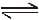Hg I42- (aq) + 2OH- (aq), which changes will decrease the equilibrium concentration of HgI42-

Detailed Solution for Chemical Equilibrium MCQ - 1 (Advanced) - Question 13

As given :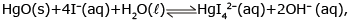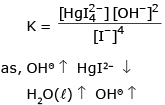*Multiple options can be correct
Chemical Equilibrium MCQ - 1 (Advanced) - Question 14

In the laboratory the equilibrium constant for a particular reaction can be measured at different temperatures. Plotting the data yields the graph shown. Which of the following statements is false?

(Note : The notation 4.40 e- 4 is equivalent to 4.40 × 10-4)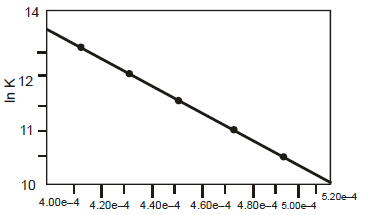Detailed Solution for Chemical Equilibrium MCQ - 1 (Advanced) - Question 14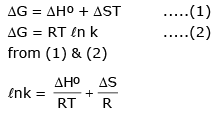slope of the line equal to ΔHº/R

*Multiple options can be correct
Chemical Equilibrium MCQ - 1 (Advanced) - Question 15

Decrease in the pressure for the following equilibria : H2O (s)H2O(l) result in the :

Detailed Solution for Chemical Equilibrium MCQ - 1 (Advanced) - Question 15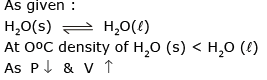∴ formation of more H2O (s) and  increase in melting point of H2O (s)

Chemical Equilibrium MCQ - 1 (Advanced) - Question 16

Statement-1 : Water boils at high temperature in pressure cooker.

Statement-2 : Increase in pressure leads to an increase in boiling point.

Detailed Solution for Chemical Equilibrium MCQ - 1 (Advanced) - Question 16

Statement-1 is true, statement-2 is true and statement-2 is correct explanation for statement-1

Chemical Equilibrium MCQ - 1 (Advanced) - Question 17

Statement-1 : A decrease in pressure leads to an increase in freezing point of water.

Statement-2 : For ice on melting volume decreases.

Detailed Solution for Chemical Equilibrium MCQ - 1 (Advanced) - Question 17

Statement-1 is true, statement-2 is true and statement-2 is correct explanation for statement-1

Chemical Equilibrium MCQ - 1 (Advanced) - Question 18

Statement-1 : The solubility of gases always increases with increase in pressure.

Statement-2 : High pressure favours the change where volume of gas decreases.

Detailed Solution for Chemical Equilibrium MCQ - 1 (Advanced) - Question 18

Statement-1 is true, statement-2 is true and statement-2 is correct explanation for statement-1

Chemical Equilibrium MCQ - 1 (Advanced) - Question 19

Statement-1 : Total number of moles in a closed system at new equilibrium is less than the old equilibrium if some amount of a substance is removed from a system.

A(g)B(g) equilibrium.

Statement-2 : The number of moles of the substance which is removed, is partially compensated as the system reached to new equilibrium.

Detailed Solution for Chemical Equilibrium MCQ - 1 (Advanced) - Question 19

Statement-1 is true, statement-2 is true and statement-2 is NOT the correct explanation for statement-1

Chemical Equilibrium MCQ - 1 (Advanced) - Question 20

Statement-1 : An exothermic reaction, non-spontaneous at high temperature, may become spontaneous at low temperature.

Statement-2 : Entropy of an exothermic reaction always decrease with decrease in temperature.

Detailed Solution for Chemical Equilibrium MCQ - 1 (Advanced) - Question 20

Statement-I is false, statement-2 is true.

Chemical Equilibrium MCQ - 1 (Advanced) - Question 21

Statement-1 : Ammonia at a pressure of 10 atm and CO2 at a pressure of 20 atm are intoduced into an evacuated chamber. If Kp for the reaction.

NH2COONH4(s)2NH3(g) + CO2(g) is 2020 atm3, the total pressure after a long time is less than 30 atm.

Statement-2 : Equilibrium can be attained from both directions.

Detailed Solution for Chemical Equilibrium MCQ - 1 (Advanced) - Question 21

Statement-1 is true, statement-2 is false.

Chemical Equilibrium MCQ - 1 (Advanced) - Question 22

In a 7.0 L evacuated chamber, 0.50 mol H2 and 0.50 mol I2 react at 427°C

H2(g) + I2 (g)2HI(g). At the given temperature, Kc = 49 for the reaction.

What is the value of Kp ?

Detailed Solution for Chemical Equilibrium MCQ - 1 (Advanced) - Question 22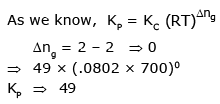Chemical Equilibrium MCQ - 1 (Advanced) - Question 23

In a 7.0 L evacuated chamber, 0.50 mol H2 and 0.50 mol I2 react at 427°C

H2(g) + I2 (g)2HI(g). At the given temperature, Kc = 49 for the reaction.

What is the total pressure (atm) in the chamber

Detailed Solution for Chemical Equilibrium MCQ - 1 (Advanced) - Question 23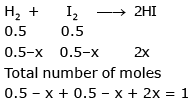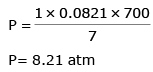Chemical Equilibrium MCQ - 1 (Advanced) - Question 24

In a 7.0 L evacuated chamber, 0.50 mol H2 and 0.50 mol I2 react at 427°C

H2(g) + I2 (g)2HI(g). At the given temperature, Kc = 49 for the reaction.

How many moles of the iodine remain unreacted at equilibrium ?

Detailed Solution for Chemical Equilibrium MCQ - 1 (Advanced) - Question 24

from above question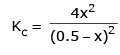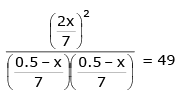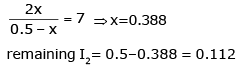Chemical Equilibrium MCQ - 1 (Advanced) - Question 25

In a 7.0 L evacuated chamber, 0.50 mol H2 and 0.50 mol I2 react at 427°C

H2(g) + I2 (g)2HI(g). At the given temperature, Kc = 49 for the reaction.

What is the partial pressure (atm) of HI in the equilibrium mixture ?

Detailed Solution for Chemical Equilibrium MCQ - 1 (Advanced) - Question 25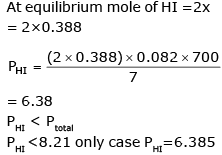## Chemistry for JEE Advanced

12 videos|53 docs|118 tests
 Use Code STAYHOME200 and get INR 200 additional OFF Use Coupon Code
Information about Chemical Equilibrium MCQ - 1 (Advanced) Page
In this test you can find the Exam questions for Chemical Equilibrium MCQ - 1 (Advanced) solved & explained in the simplest way possible. Besides giving Questions and answers for Chemical Equilibrium MCQ - 1 (Advanced), EduRev gives you an ample number of Online tests for practice

## Chemistry for JEE Advanced

12 videos|53 docs|118 tests